$\def\SplitInversions{ {\mathrm{SplitInversions}} } \def\lcs{ {\mathrm{lcs}} }$

## Example Problem Solutions

Problem 1 (graph distance and spanning trees). Consider the following weighted graph: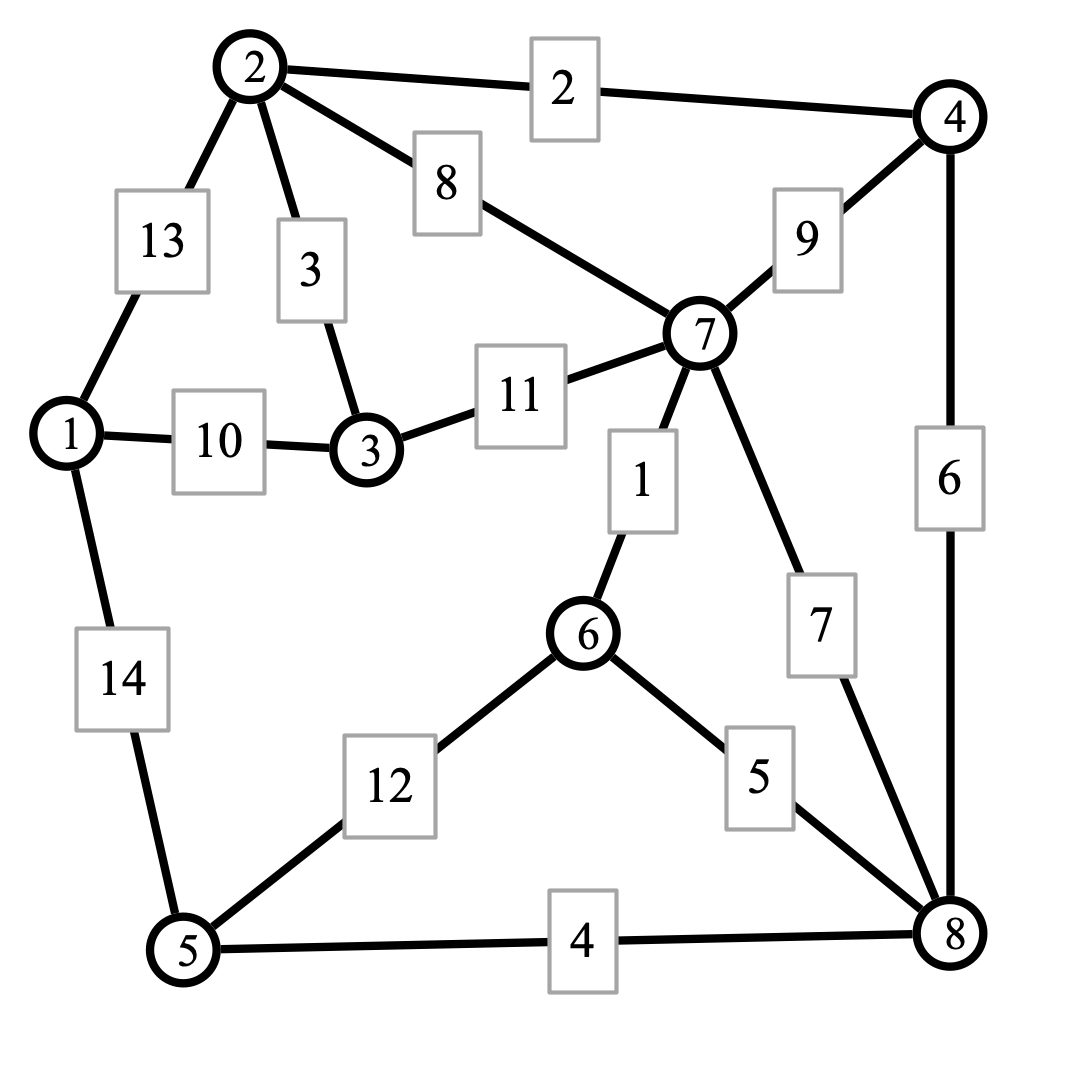1. Apply Dijkstra’s algorithm to find the distance of every other vertex from vertex 1. Also indicate the shortest paths from 1 to each other vertex. (Note that the shortest paths form a spanning tree.)

2. Apply your favorite algorithm to find a minimum spanning tree (MST) for the graph.

Solution. Here are the shortest paths from vertex 1. Notice that vertices are now labeled with distances.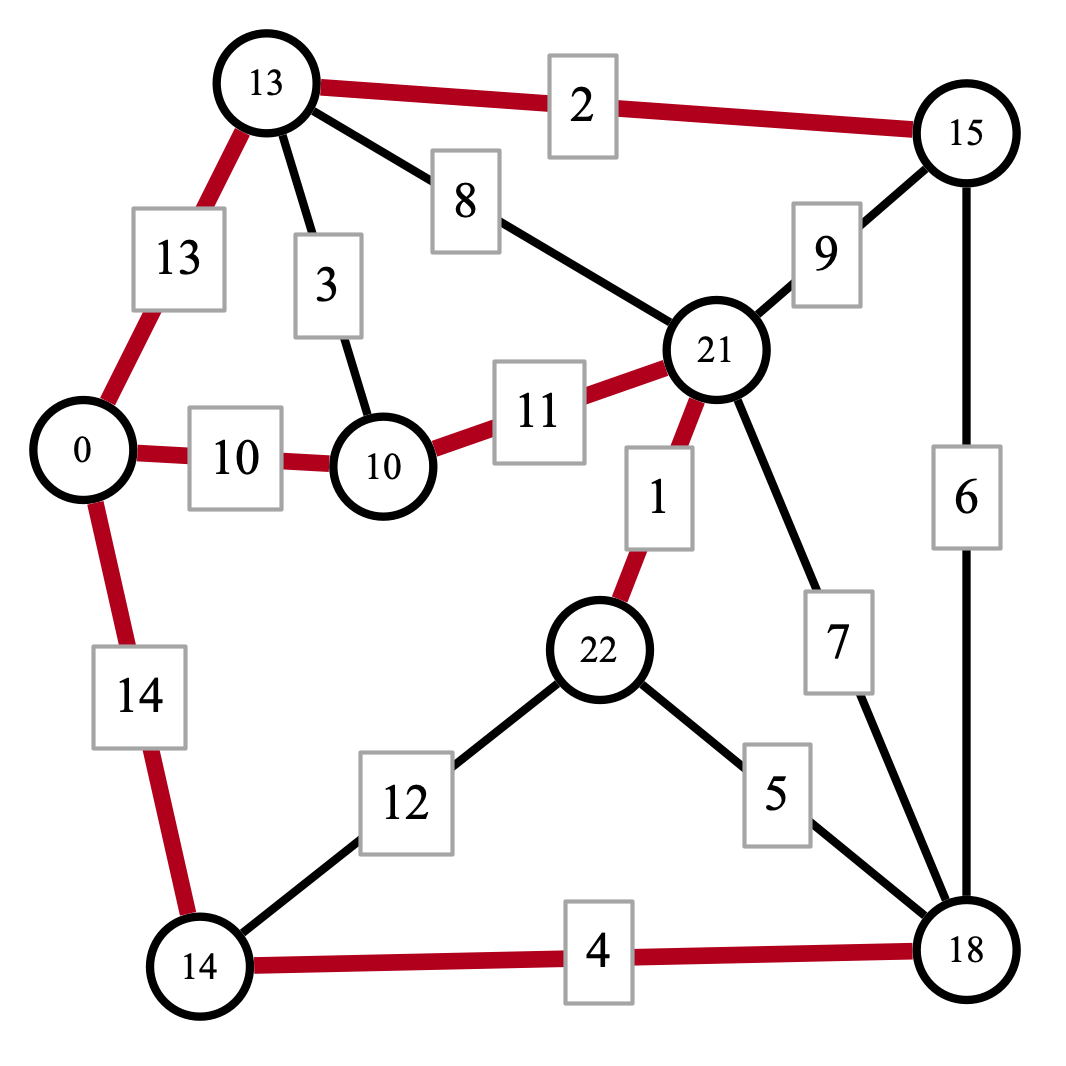Here is an MST for the original graph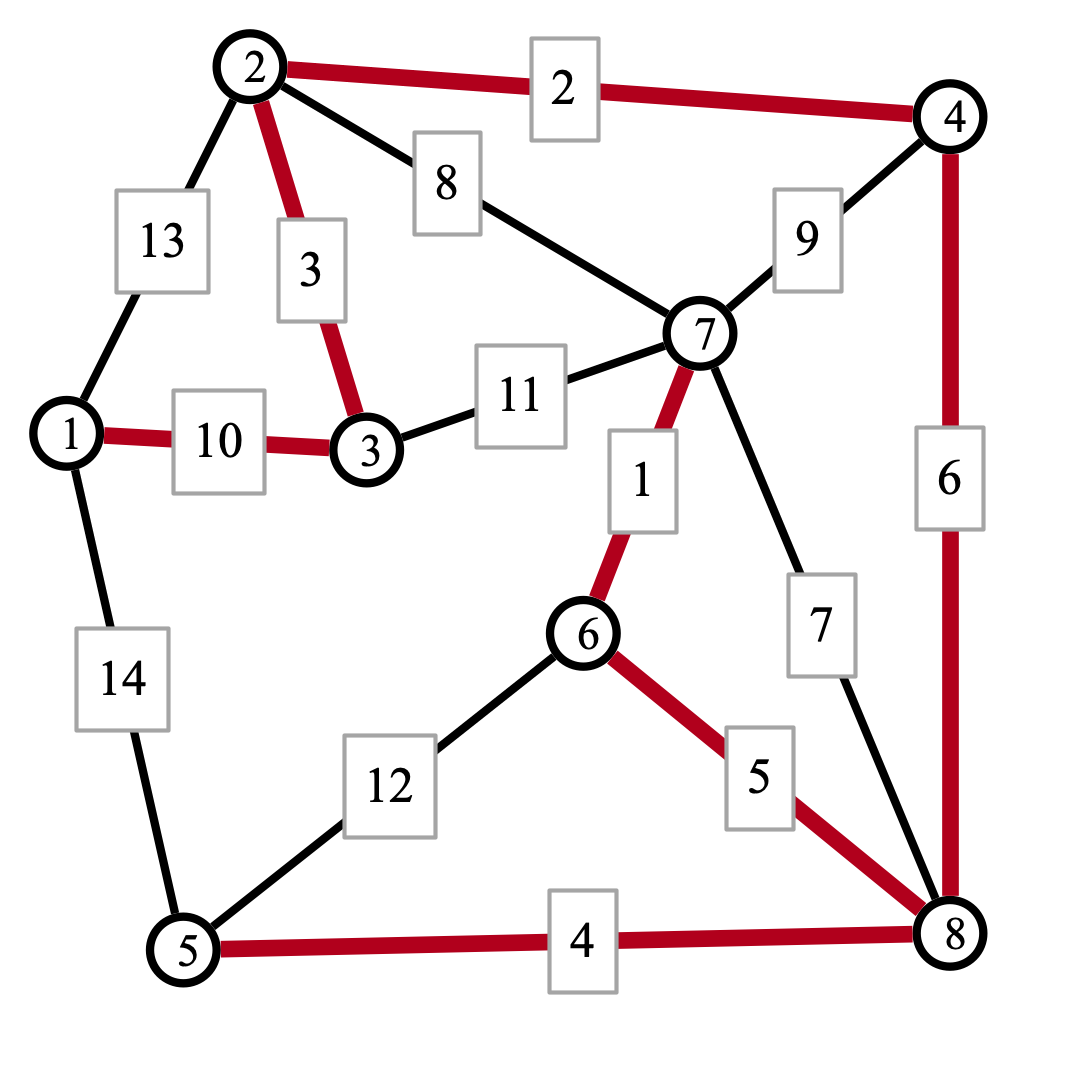Problem 2 (counting inversions). Suppose $$a$$ is an array of integers of size $$n$$. Recall that two indices $$i, j$$ are an inversion if $$i < j$$ and $$a[i] > a[j]$$. It is straightforward to count the total number of inversions in an array in time $$O(n^2)$$ by iterating over all pairs of indices $$(i, j)$$ and checking if each is an inversion.

Suppose you are given a method $$\SplitInversions(a, r, s, t)$$ that, given an array $$a$$ and indices $$r, s, t$$ satisfying $$r < s < t$$, computes the number of inversions $$(i, j)$$ in $$a$$ with $$r \leq i < s$$ and $$s \leq j < t$$, and whose running time is $$O(t - r)$$. Design a divide and conquer algorithm that uses $$\SplitInversions$$ as a subroutine to compute the number of inversions in $$a$$. Use the master theorem to compute the running time of your procedure (which should be substantially faster than $$O(n^2)$$.

Solution. If we would like to count inversions of $$a$$ between indices $$r$$ and $$t$$ (i.e., inversions in $$a[r..t]$$, there are three possibilities for each inversion $$(i, j)$$:

1. $r \leq i, j < s$
2. $s \leq i, j \leq t$
3. $r \leq i < s \leq j \leq t$

We can devise an algorithm that counts inversions as follows: recursively count inversions of satisfying 1 and 2 above, and use $$\SplitInversions$$ to count the inversions of type 3. Here’s the pseudocode:

1
2
3
4
5
6
7
8
# count inversions of a of the form (i, j) with r <= i < j <= t
CountInversions(a, r, t):
if t - r <= 1 then return 0
s <- (t + r) / 2
left <- CountInversions(a, r, s-1)
right <- CountInversions(a, s, t)
split <- SplitInversions(a, r, s, t)
return left + right + split


For the running time analysis, we can apply the master theorem. The recursive calss break the problem down into $$2$$ subproblems of size $$n / 2$$, so we have $$a = b = 2$$. The running time of the method (not counting the recursive calls) is $$O(n)$$, so we are case 2 of the master theorem. Thus the overall running time is $$O(n \log n)$$.

Problem 3 (longest common subsequences). Suppose $$a$$ and $$b$$ are arrays of length $$n$$ and $$m$$, respectively. We say that $$a$$ and $$b$$ have a common subsequence of length $$k$$ if there are indices $$1 \leq i_1 < i_2 < \cdots < i_k \leq n$$ and $$1 \leq j_1 < j_2 < \cdots < j_k \leq m$$ such that $$a[i_1] = b[j_1], a[i_2] = b[j_2], \ldots, a[i_k] = b[j_k]$$. For example, if $$a = [A, L, G, O, R, I, T, H, M]$$ and $$b = [A, M, H, E, R, S, T]$$, then $$A, R, T$$ is a common subsequence of $$a$$ and $$b$$ of length 3.

Use dynamic programming to devise an algorithm that computes the length of the longest common subsequence of two arrays in time $$O(n m)$$.

Hint. Suppose $$a$$ and $$b$$ are given. For $$i \leq n$$ and $$j \leq m$$, let $$\lcs(i, j)$$ be the length of the longest common subsequence of $$a[1..i]$$ and $$b[1..j]$$. How does $$\lcs(i, j)$$ relate to other values of $$\lcs$$? What if $$a[i] = b[j]$$? Or if $$a[i] \neq b[j]$$?

Solution. We begin by writing a recursion relation that relates $$\lcs(i, j)$$ to values of $$\lcs(i', j')$$ with $$i' \leq i$$ and $$j' \leq j$$. In this case, there are two possibilities to consider: (1) $$a[i] = b[j]$$, and (2) $$a[i] \neq b[j]$$. In the first case $$a[i]$$ and $$b[j]$$ can contribute to the longest common subsequence, while in the second case they cannot. We can express the relationship recursively as

$\lcs(i, j) = \begin{cases} 0 &\text{ if } i = 0 \text{ or } j = 0\\ \max(\lcs(i-1, j), \lcs(i, j-1)) &\text{ if } a[i] \neq b[j]\\ \max(1 + \lcs(i-1, j-1), \lcs(i-1, j), \lcs(i, j-1)) &\text{ if } a[i] = a[j] \end{cases}$

To turn this recursion relation into a dynamic programming solution, we can store the values of $\lcs(i, j)$ in a two dimensional array. Here’s pseudocode:

1
2
3
4
5
6
7
8
9
10
11
12
13
14
LCS(a, b):
lcs <- 2d array
for i from 0 to n set lcs[i, 0] <- 0
for j from 0 to m set lcs[0, j] <- 0
for i from 1 to n
for j from 1 to m
if a[i] = b[j] then
lcs[i, j] <- Max(1 + lcs[i-1, j-1], lcs[i-1,j], lcs[i,j-1])
else
lcs[i, j] <- Max(lcs[i-1,j], lcs[i,j-1])
endif
endfor
endfor
return lcs[n, m]


Problem 4 (Ford-Fulkerson computation). Consider the following directed weighted graph $$G$$ with source $$s$$ and sink $$t$$: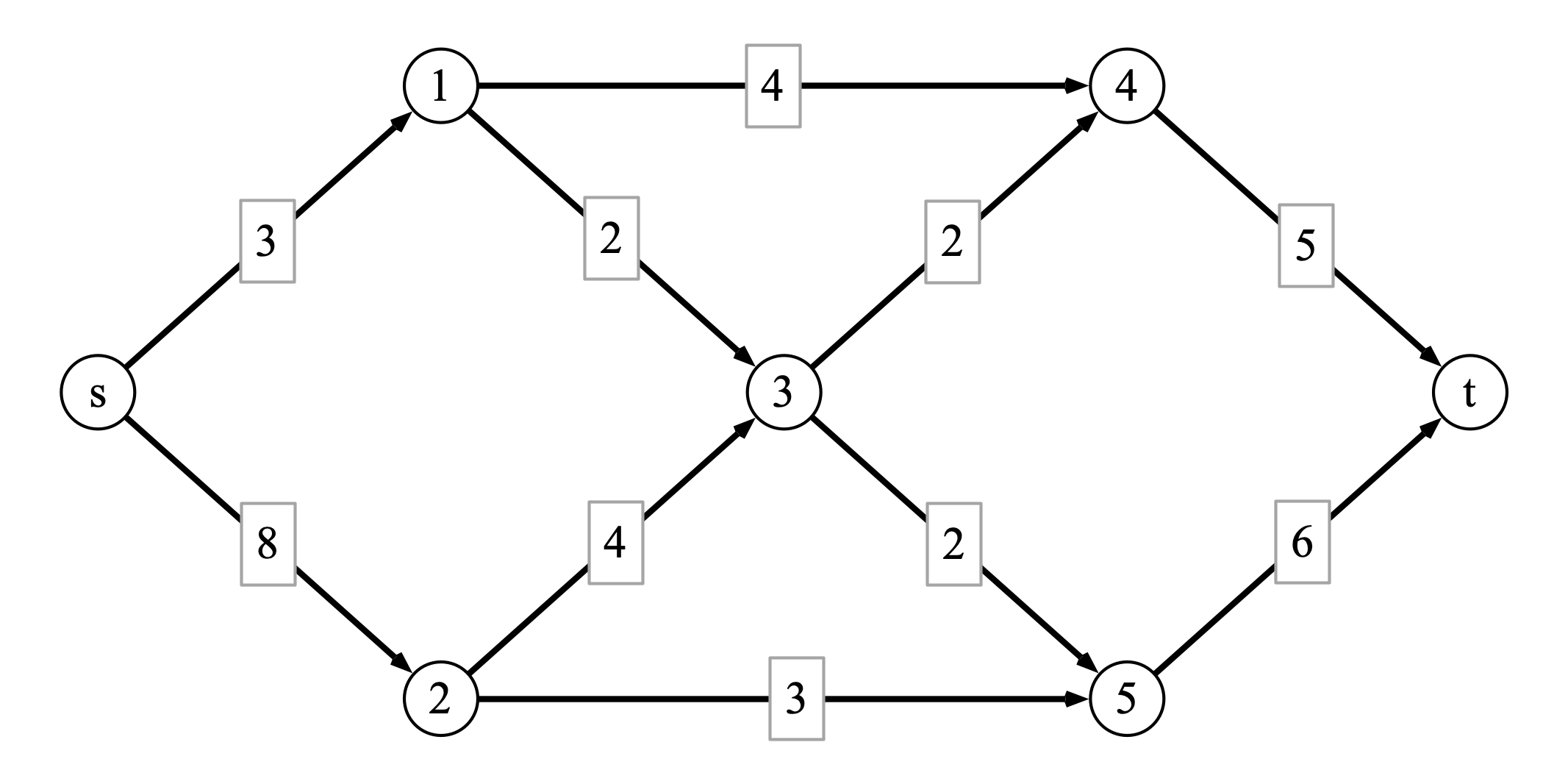At some intermediate step of executing the Ford-Fulkerson algorithm, you find the flow $$f$$ defined as follows: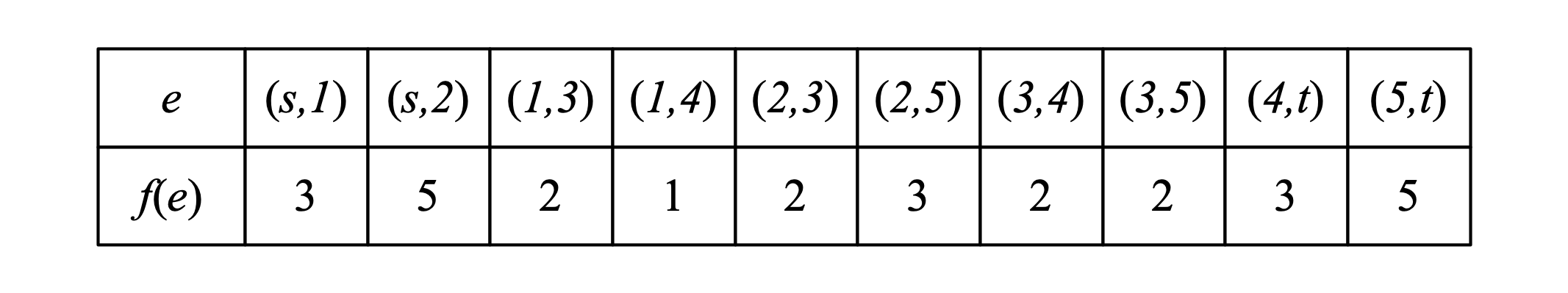The following questions refer to the process of completing an execution of Ford-Fulkerson on the graph.

1. What is the value of the flow $$f$$ defined above?
2. Draw the residual graph $$G_f$$ resulting from the flow $$f$$ on $$G$$.
3. Find an augmenting path for the graph $$G_f$$.
4. Write the flow resulting from routing additional flow through the augmenting path you found in step 3.
5. Argue that the flow you found in part 4 is a maximum flow.

Solution.

The value of the given flow is $$8$$. Here is the residual graph, together with an augmenting path indicated in red.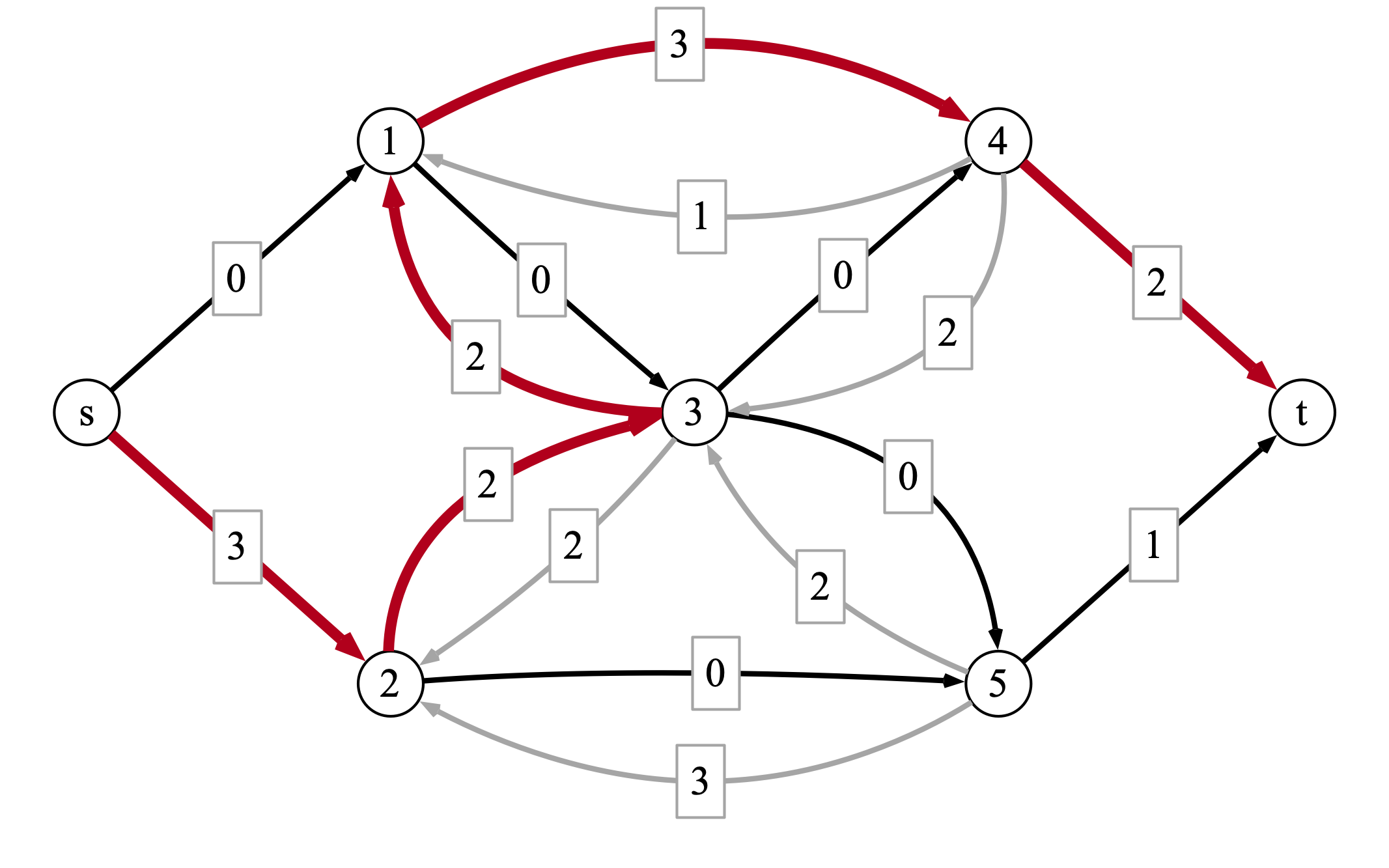After routing two additional units of flow across the augmenting path, we arrive at the following flow: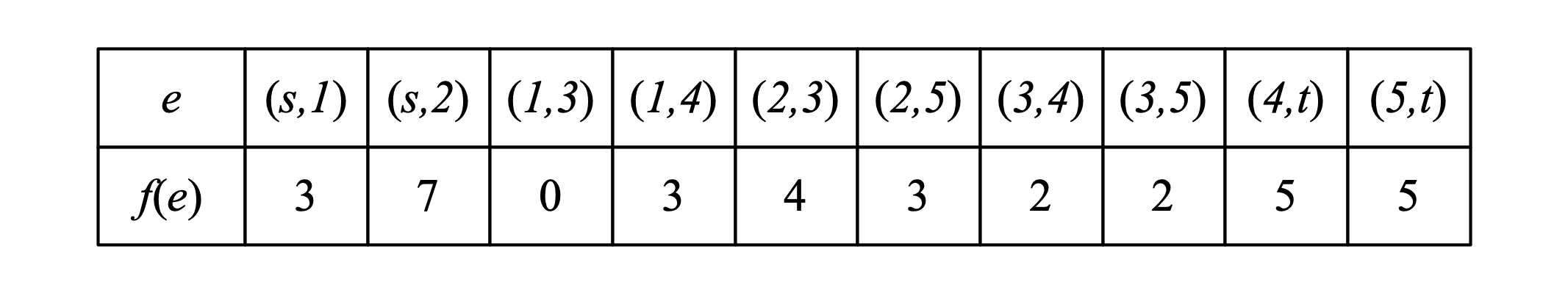With this flow, the residual network becomes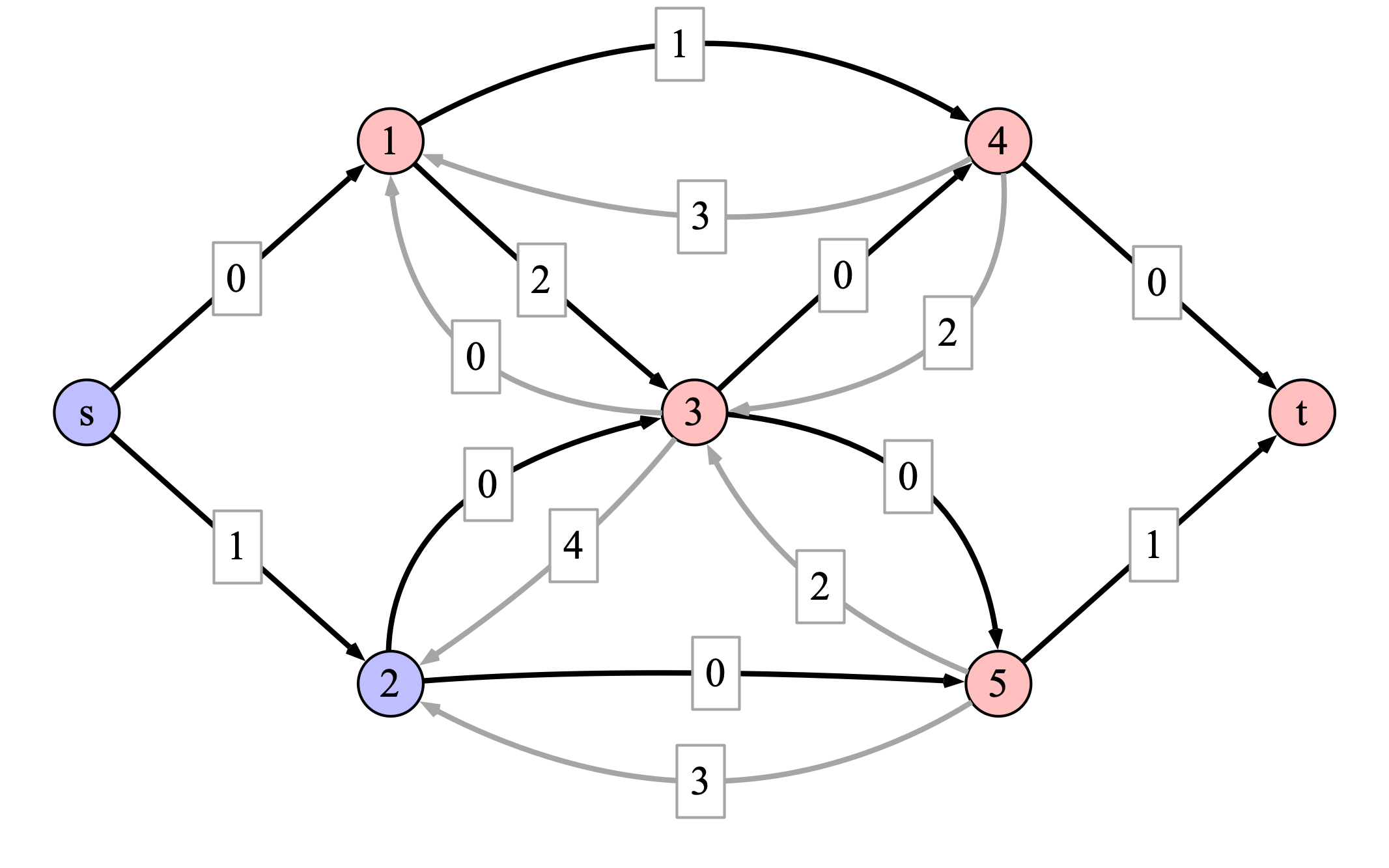In this case there are no augmenting paths from $$s$$ to $$t$$. The blue nodes are all nodes reachable from $$s$$, while the red nodes are not. Looking at the original graph, we can see that the blue-red partition corresponds to a cut of capacity 10. Since the updated flow has value 10, there is no larger flow possible, so we’ve found a maximum flow.

Problem 5 (MaxFlow reduction). After college, three friends, Alice, Bob, and Carol, decided to move to the city and live together as roommates. In order to save time and money, they decide that every night except Saturdays, one of them will cook for everyone in the apartment. In order to do things equitably, they would like to come up with a schedule in which each person cooks dinner two nights a week. The roommates lead busy lives, however, and they are not available to cook every night: Alice is only able to cook on Mondays, Wednesdays, and Fridays; Bob is only availble on Tuesdays, Thursdays, and Fridays; Carol can only cook on Wednesdays, Fridays, and Sundays.

1. Draw a bipartite graph that indicates the three roommates’ availabilities to cook.
2. Given the graph of part 1, how could you determine if there is a cooking schedule such that each roommate cooks two nights a week, and someon cooks every night (except Saturdays).
3. How could you transform the graph from 1 into an instance of MaxFlow such that finding a maximum flow will either reveal a cooking schedule as in part (2) or indicate that no such schedule exists?

Solution. For part 1, here is a bipartite graph indicating availabilities: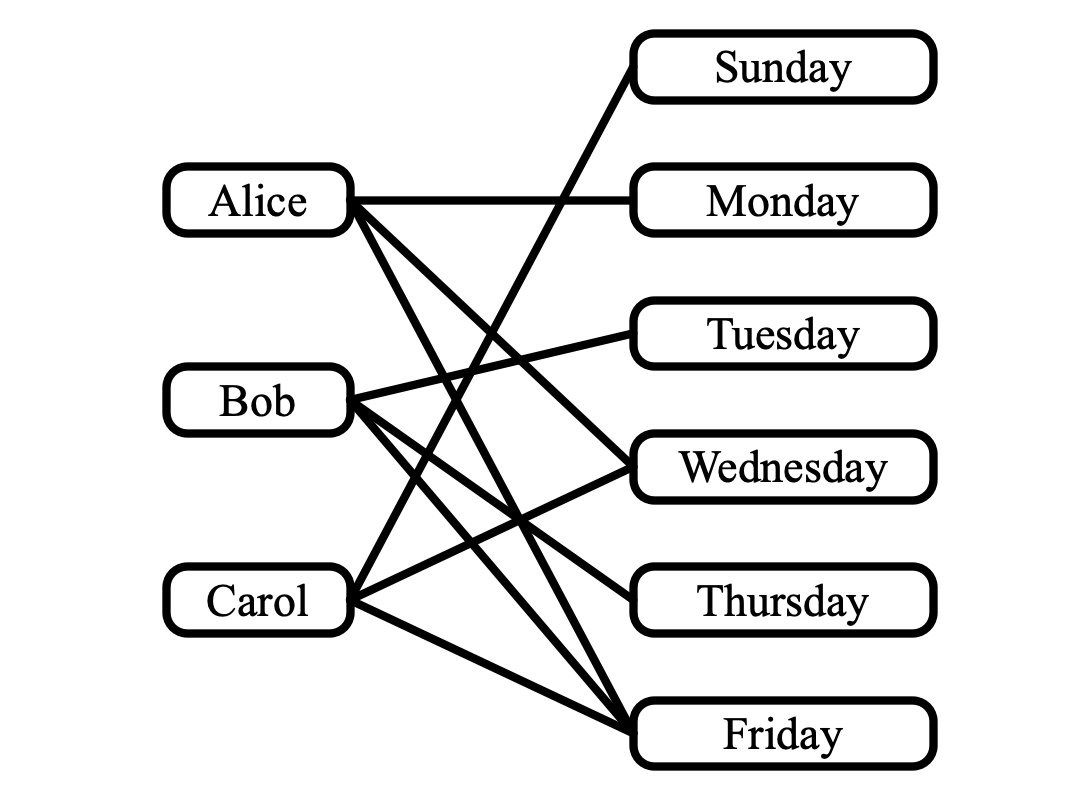For part 2, a cooking schedule corresponds to a set of edges in the graph depicted above such that (1) every person is incident to two edges, and (2) every day is incident to a single edge. For part 3, here is a corresponding flow network: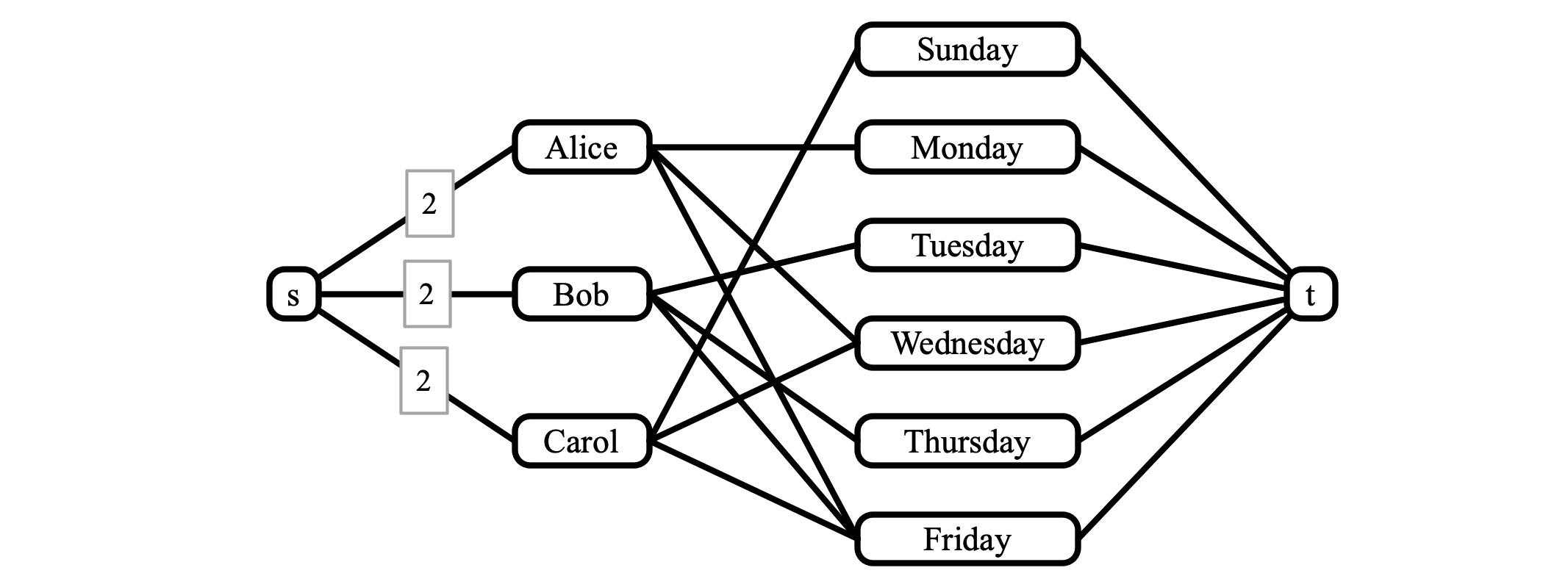All edges are directed from left to right, and all edges have capacity 1, except those whose capacities are indicated as 2. Observe that there is a cooking schedule as required if and only if the flow network admits a maximum flow of value 6. In this case, a flow of value 6 must route 2 units of flow to each person. Since the outgoing edges from each person have capacity 1, if two units of flow are going to a person, then the two units of flow must be directed away from each person across two edges (corresponding to the person’s assigned days). On the other hand, the capacity constraint of 1 out of each day to the sink $$t$$ ensures that only one person is assigned to each day.

Problem 6 (NP completeness). In a film class at Cook-Levin University, the $$m$$ students are asked to list a few of their favorite movies for a list of “The $$n$$ Greatest Movies of All Time,” (for some value of $$n$$). A semester lasts $$k$$ weeks, and the class can only screen a single film each week. Your task is to determine whether or not it is possible to choose a selection of $$k$$ movies such that at least one movie from each student’s list is screened at some point in the semester. Show that your task is NP-complete.

Solution. As always, showing NP-completeness requires us to show (1) that the problem is in NP, and (2) a reduction from some NP-complete problem to our problem.

To see that the movie selection problem is in NP, we must describe a polynomial time verifier for the problem. In this case a certificate can consist of a list of $$k$$ movies—the schedule of movies that will be screened. Given such a certificate, a verifier iterates over the students’ lists and checks that some movie from each list appears on the schedule. The verifier accepts the certificate if this is the case, and rejects the certificate otherwise. This procedure can be implemented to run in polynomial time in the input size (an elementary procedure could take time $$O(n m k)$$).

To show the problem is NP complete, we must describe a reduction from an NP-complete problem to the scheduling problem. To this end, we give a reduction from vertex cover (VC). Recall that the input to VC consists of a graph $$G = (V, E)$$ and a number $$k$$. The output is “yes” if $$G$$ admits a vertex cover—i.e. a set $$C$$ of vertices such that every edge has at least one endpoint in $$C$$—of size $$k$$.

Given an instance $$G = (V, E), k$$ of VC, we can form an instance of the movie scheduling problem as follows: associate each vertex $$v \in V$$ with a movie. For each edge $$e = (u, v) \in E$$, create a student who’s movie list consists of movies $$u$$ and $$v$$. Now, a schedule of $$k$$ movies satisfies the requirement that at least one movie is chosen from each student’s list if and only if the schedule corresponds to a vertex cover for $$G$$. Thus, this correpsondence gives a reduction from VC to the movie scheduling problem.